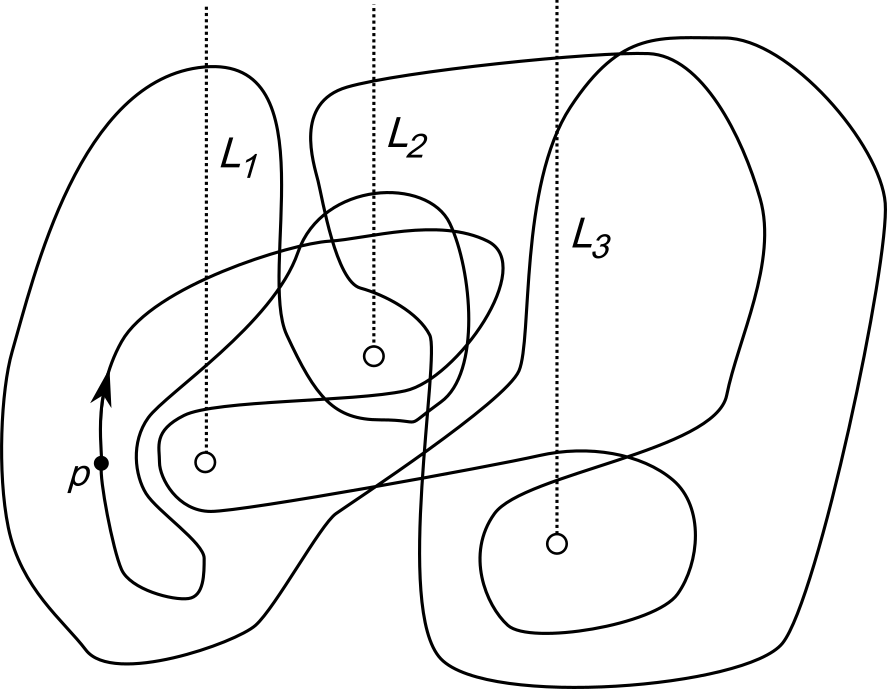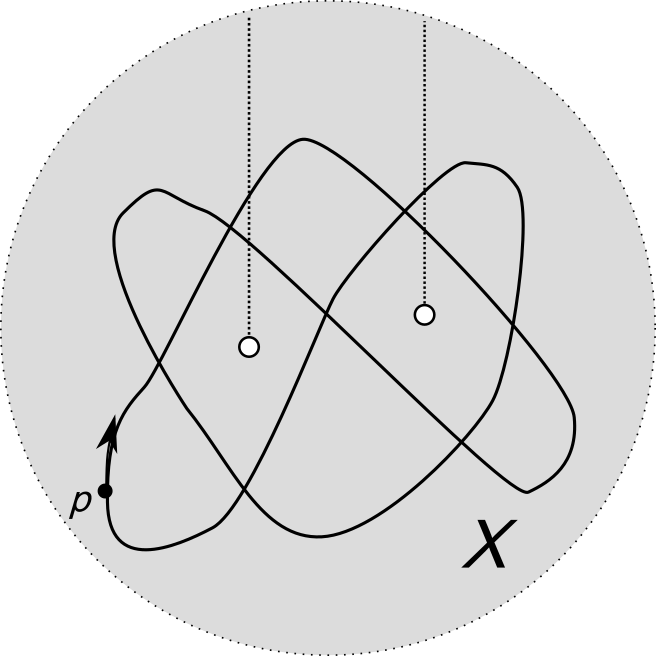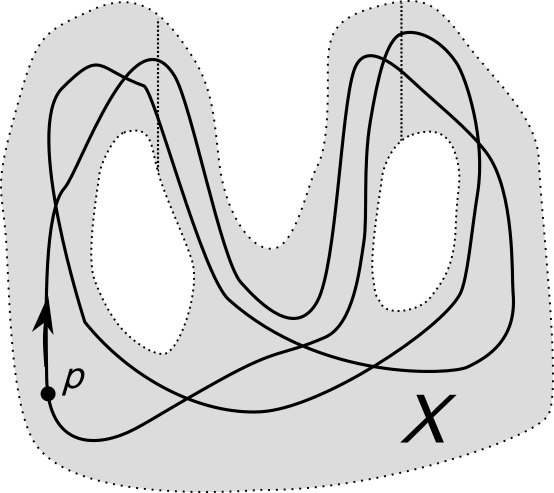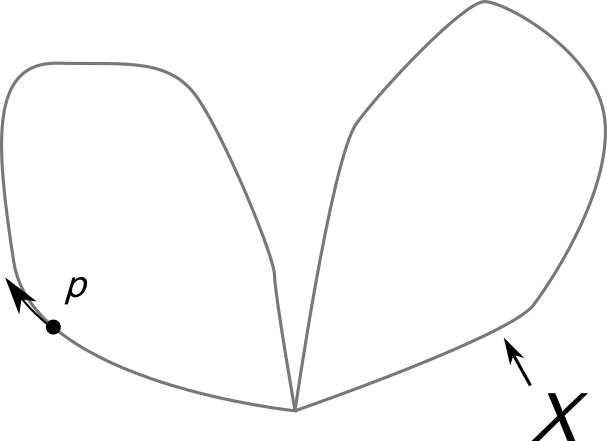# RUBBER - Editorial

Practice

Contest

Author: Jineon Baek

Tester: Suchan Park

Editorialist: Jineon Baek

Very Hard

# PROBLEM:

You are given a polyline and n points. You can think the given polyline as a rubber band, so it can be stretched freely,
but you are not allowed to cut into pieces. Is it possible to move the rubber band so that the band escape from all the points?

### EXPLANATION:

First of all, let’s assume that the positions of nails have different x coordinates. This can be achieved by, say, rotating the whole plane by a small angle.

We can check if the rubber band can be separated by the following method: For the i'th nail from left, assign a ray L_i directed upward from the nail. Now pick any point p on the rubber band and move p along it. Whenever the point p passes L_i from left to right, assign a number i, and whenever p passes L_i from right to left, assign a number -i. Call the final sequence we obtain by moving p along the rubber band completely as w.[Fig 1]: An example of 3 nails and a rubber band, with the sequence w = (something) assigned to the band.

The rubber band can be freed if and only if we can get an empty sequence by repeatedly remove two adjacent numbers of same absolute value and opposite sign. For example, the sequence `5 -2 2 4 1 3 3 -3 -3 -1 -4 -5` corresponds to a removable band, but the sequence `1 2 -1 -2` isn’t. The algorithm can be implemented effectively by examining all possible intersections in order, and it takes O(NM) time.

It remains to show why this method works. Note that we can first assume that the rubber is on the set X, which is the plane with the points corresponding to nails removed. The rubber band can be freed if and only if the rubber band can be moved inside the set X and be condensed to a point. If this is possible, we say that the rubber is null-homotopic in X.
We can deform X into a collection of circles joined along a single common point as the following picture. Note that the rubber band deforms along too. Noe that this deformation process does not change the answer to the problem of whether the band is null-homotopic or not. This is something we call homotopy equivalence in mathematics.[Fig 2]: A sequence of pictures deforming the plane with nails removed to a collection of circles joined along a single common point.

Each nail makes a hole, and thus corresponds to one circle in the final picture. By the way how we deformed X, we can see that the sequence we defined in previous paragraph tracks down the sequence of circles the rubber band wraps around: the absolute value of each number denotes the circle the rubber band wraps around, and the sign denotes the direction (clockerwise/counterclockerwise) of the rubber band.
The fact that the rubber band is null-homotopic if and only if the sequence can be reduced to an empty sequence can be shown rigorously by the fact that the fundamental group of a wedge product of circles is a free group. Still, even without this mathematical fact, you can convince yourself that the method above will work by playing around with a couple examples.

### AUTHOR’S AND TESTER’S SOLUTIONS:

Author’s solution can be found here.

Tester’s solution can be found here.

1 Like